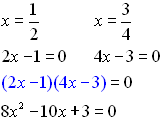Factoring

The graphing calculator can be a tremendous help when attempting to multiply or factor algebraic expressions.  There are several approaches you may use depending upon the known information.

Answers can be "checked" to see if the correct factors have been found:

(particularly helpful when dealing with multiple choice questions)

 Check Method 1:  Check factors/answer on the home screen in equation form.                                       Factor x2 - 12x + 36.    OR      Find (x - 6)2.
 Choose your "favorite" positive one-digit (for ease) integer value and store the value in x (do not pick 0 or 1).   For example, to store a 7:   7  STO► x Hit ENTER.  Enter the problem to be factored (or multiplied) and set "="  to one of the possible answers (or the answer you want to check).  The "=" sign is under 2nd MATH (TEST) #1 =. Hit ENTER. If a 0 appears, this is NOT the correct answer. If a 1 appears, this IS the correct answer.Check Method 2:  Check factors/answer by graphing.                                       Factor x2 - 12x + 36.    OR      Find (x - 6)2.
 Enter the expression into the Y1= on the calculator. Enter the possible answer response into Y2=. Move to the left of the Y2= and choose the open circle.  This setting will allow you to clearly see the animated circle crossing the screen. When the answer is correct, the animated circle will be "riding" on top of the original graph. Keep checking until the correct answer is found. The only difficult feature of this method is the occasional need to adjust the viewing window to see the graph.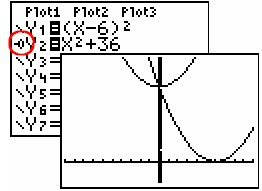The animated bubble does not run along the  original curve.  It forms its own graph.  This is not the correct solution.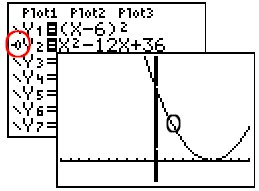The animated bubble runs along the original graph.  This is the answer.

Answers can be "found" by graphing and finding the zeros of an equation.

 Graph Method:  Factors can be found by finding the zeros of an equation.                                    Find the factors of x2 - 3x - 4.
• Enter the expression to be factored into Y=.
• Graph.  The window may need to be adjusted to see where the graph crosses the x-axis (the x-intercepts or zeros).
• Use the ZERO (2nd CALC #2:zero) function to find the x-intercepts or zeros (also called the roots).  See steps below: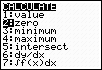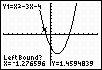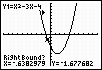2nd CALC to locate ZERO Move spider to left of  x-intercept. ENTER. Move spider to right of x-intercept.  ENTER.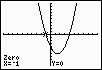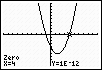We know have the zeros, x = -1 and x = 4. When asked for Guess, hit ENTER. Write down the x = -1 Repeat the process to find the other x-intercept. Write down x = 4.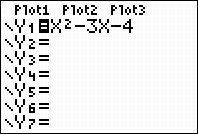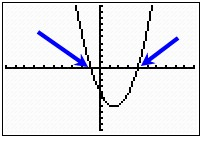The roots (zeros) of this equation
(where it crosses the x-intercept)
will help us find the factors.

 Using the zeros (roots), we can find the factors.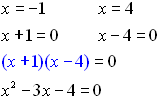Check to see if the factors are correct.  Multiply the factors to see if they give you the original expression. ANSWER:   Factors of x2 - 3x - 4 = 0 are (x + 1)(x - 4)

 Example 1:  (Multiple choice question) The factors of x2 + 5x +6 are      (1) (x + 1)(x + 6)      (2) (x + 3)(x + 2)      (3) (x + 5)(x + 1)      (4) (x + 6)(x - 1) Method 1: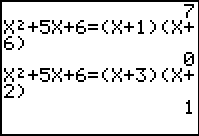The "favorite" number stored was 7. When you hit ENTER and a "1" appears, you have found the answer. Method 2: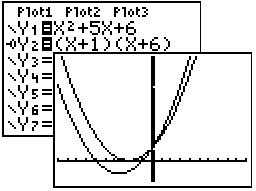The two graphs do not overlap. This is not the answer.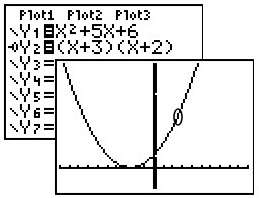The animated bubble overlaps the original graph.  This is the answer. Example 2:  (Multiple choice question) The room that is shown in the figure below has a floor space of 2x2 + x - 15 square feet.  If the width of the room is (x + 3) feet, what is the length?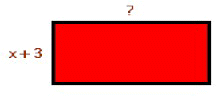(1) (x - 3)      (2) (2x - 5)      (3) (x - 5)      (4) (2x + 5) Method 1:Answers are tested until the correct answer is found. OR Check your answer to be sure you chose wisely.

Method 2: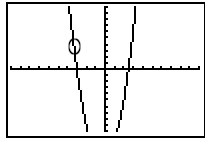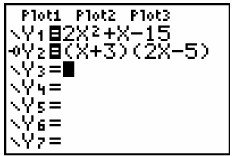Example 3:  (Free response question)
Find the factors of  8x2 - 10x + 3.

This is a tough one, but you can do it.
Find the zeros and then form the factors.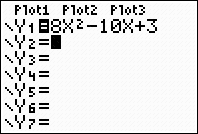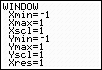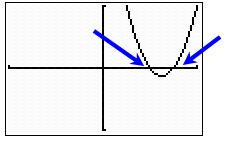Find the zeros (x-intercepts).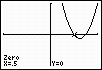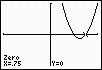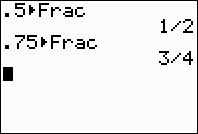If necessary, convert the
decimal answers to fractions.
MATH - #1Frac

Now, form the factors.